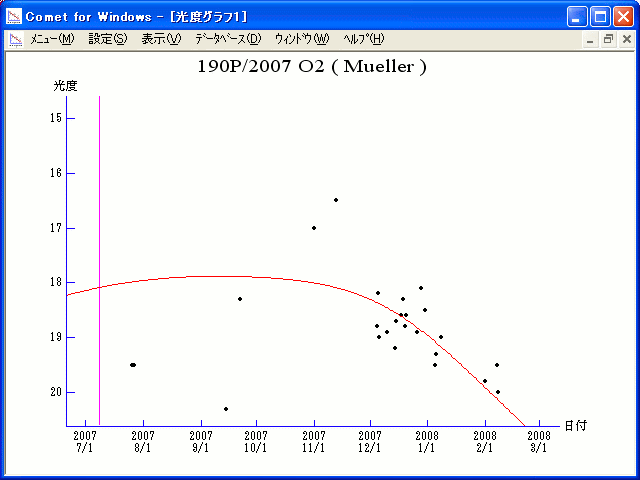# \$B%_%e%i!

190P/Mueller (2007)

 English version Home page Updated on April 6, 2008###\$B%W%m%U%#!<%k(B

 \$BId9f(B 190P/2007 O2 \$B8!=PF|(B 2007\$BG/(B7\$B7n(B26\$BF|(B \$B8!=P8wEY(B 19.5\$BEy(B \$B8!=P L. Buzzi and F. Luppi (Varese, Italy)

###\$B###\$B50F;MWAG(B

```                    Epoch = 2007 June 29.0 TT
T = 2007 July  8.20087 TT        Peri. =  49.73116
e = 0.5206516                    Node  = 336.11998 2000.0
q = 2.0319152 AU                 Incl. =   2.18993
a =  4.2389107 AU   n = 0.11293347   P =   8.73 years
```

###\$B8wEYJQ2=(B

```        m1 = 10.0 + 5 log\$B&\$(B + 20.0 log r
```##### \$B50F;MWAG\$O!"(B IAUC 8859 \$B\$K7G:\\$5\$l\$?\$b\$N\$G\$9!#(B \$B8wEY%0%i%U\$O(BComet for Windows\$B\$G:n@.\$7\$?\$b\$N\$G\$9!#(B